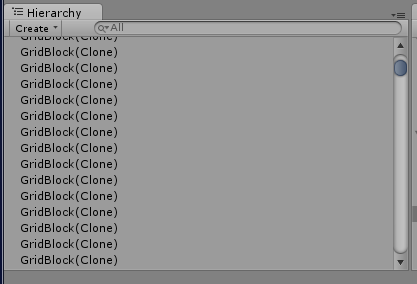# Saving lots of objects

I know this is a hard one so a big thanks in advance. So I have this game called borda and the world is made of many many cubes. I can’t figure out how to save it all with out the game crashing. So if you guys can help me have unity save and load all this stuff I would be very thankfull.

This is how it looksSounds like you’ll want to use custom data saving with a Coroutine. Something along the lines of this:

``````using UnityEngine;
using System.Collections;
using System.IO;

public class SaveGame : MonoBehavior
{
public GameObject[] cubes;
protected string savename = "savegame";

private void Start()
{
cubes = FindGameObjectsWithTag("Floor");
}

public IEnumerator Save()
{
foreach(GameObject cube in cubes)
{
Vector3 pos = cube.transform.position;
Quaternion rot = cube.transform.rotation;

yield return new WaitForSeconds(0.01f);
}
}

public void AddCube(Vector3 pos, Quaternion rot)
{
float[] xyz = new float, hpb = new float;
// Pos                 Rot
xyz = pos.z; xyz = pos.y; xyz = pos.z;
pos = rot.z; pos = rot.y; pos = rot.z;

if(File.Exists("saves/" + savename + ".txt"))
{
using(StreamReader saveFile = File.OpenText("saves/" + savename + ".txt"))
{
foreach(float item in xyz)
{
saveFile.Write(item.ToString("R"));
}
saveFile.Write("-"); // Separator
foreach(float item in hpb)
{
saveFile.Write(item.ToString("R"));
}
saveFile.Write("
``````

“); // Line break
}
}else{
using(StreamWriter saveFile = File.CreateText(“saves/” + savename + “.txt”))
{
foreach(float item in xyz)
{
saveFile.Write(item.ToString(“R”));
}
saveFile.Write(”-“); // Separator
foreach(float item in hpb)
{
saveFile.Write(item.ToString(“R”));
}
saveFile.Write(”
"); // Line break
}
}
}
}

Now, prewarning: I didn’t have a computer with Unity to test this to make sure it will work, but you can probably take it from here and fix it up to work how you want it to.